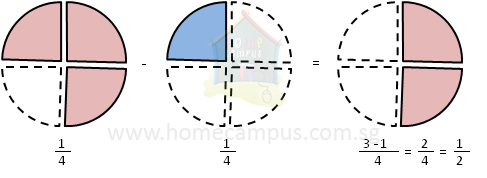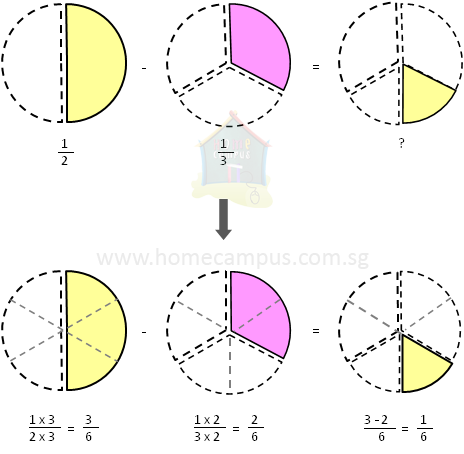## Subtracting Like and Unlike Proper Fractions

Practice Unlimited Questions

#### How to subtract like fractions?

Step 1: Subtract all the numerators. (Denominator will remain the same.)
Step 2: Simplify if required.

See the example below

#### How to subtract unlike fractions?

Step 1: Change unlike fractions to like fractions. i.e. find a common denominator.
Step 2: Subtract all the numerators.
Step 3: Simplify if required.

See the example below

#### 1. The video below explains how to subtract proper fractions.

A proper fraction is a fraction in which the numerator is smaller than the denominator.

#### 2. Monica had    1 4 of a pie and Sarah had    3 4 of the pie. How much more of the pie had Sarah than Monica?

Sarah  =>
 3 4

Monica  =>
 1 4

Sarah  −  Monica  =>
 3 4
−
 1 43 4
−
 1 4

 3 - 1 4

 2 4

 2 / 2 4 / 2
(simplify)
 1 2

 1 2
a pie more than Monica.

#### 3. Elly gives    1 2 of a pizza to Charlie and    1 3 of the pizza to Derek. How much more of the pizza does Elly give to Charlie than Derek?

Charlie  =>
 1 2

Derek  =>
 1 3

Charlie  −  Derek  =>
 1 2
−
 1 3Both Charlie and Derek had 1 slice each but Charlie's slice was bigger than Derek's slice because Charlie's pizza was cut into 2 while Derek's pizza was cut into 3.

To compare the two slices, first cut both pizzas into equal number of slices.

 1 2
−
 1 3

 1 x 3 2 x 3
−
 1 x 2 3 x 2

 3 6
−
 2 6
(changed the denominator to 6)
 1 6

Elly gives
 1 6
of a pizza more to Charlie than to Derek.
Since the two fractions,
 1 2
and
 1 3
are unlike fractions (as the denominators are different), we must first change them to like fractions before we can subtract them (that is, find a common denominator).